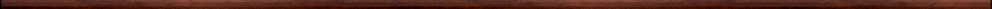Maple worksheets on the numerical evaluation of mathematical functionsNumerical methods topics:

They are all compatible with Classic Worksheet Maple 10.A procedure for calculating square roots - sqrt.mws

• Newton's method for calculating square roots.
• Hero's formula for calculating square roots.
• Starting approximation for a general square root procedure.
• A procedure for calculating the square root of a number: squareroot.

Evaluating the sine function from its Maclaurin series - sine_MS.mws

• Graphing partial sums of the Maclaurin series for sin(x).
• A procedure for evaluating the sine function from its Maclaurin series.
• Argument reduction.
• An arbitrary precision procedure for evaluating the sine function.

Evaluating the cosine function from its Maclaurin series - cosine_MS.mws

• Graphing partial sums of the Maclaurin series for cos(x).
• A procedure for evaluating the cosine function from its Maclaurin series.
• Argument reduction.
• An arbitrary precision procedure for evaluating the cosine function.

Evaluating the exponential function from its Maclaurin series - exp_MS.mws

• Graphing partial sums of the Maclaurin series for exp(x).
• A procedure for evaluating the exponential function from its Maclaurin series.
• Argument reduction.
• An arbitrary precision procedure for evaluating the exponential function.

Evaluating the natural logarithm function from its Maclaurin series - natlog_MS.mws

• Graphing partial sums of the Maclaurin series for ln(1+x).
• A procedure for evaluating the natural logarithm function from a Taylor series.
• Argument reduction.
• An arbitrary precision procedure for evaluating the natural logarithm function.

Evaluating the inverse tangent function from its Maclaurin series - arctan_MS.mws

• Graphing partial sums of the Maclaurin series for arctan(x).
• A procedure for evaluating the inverse tangent function from its Maclaurin series.
• Argument reduction.
• An arbitrary precision procedure for evaluating the inverse tangent function.

Evaluating the inverse sine function from its Maclaurin series - arcsin_MS.mws

• Graphing partial sums of the Maclaurin series for arcsin(x).
• A procedure for evaluating the inverse sine function from its Maclaurin series.
• Argument reduction.
• An arbitrary precision procedure for evaluating the inverse sine function.

Procedures for evaluating the sine function - sine.mws

• A fixed (low) precision procedure for evaluating the sine function.
• A general procedure for evaluating the sine function: sine.

Procedures for evaluating the sine and cosine functions - sincos.mws

• Arbitrary precision procedures for evaluating the sine and cosine functions.
• Fixed precision procedures for evaluating the sine and cosine functions.
• General procedures for evaluating the sine and cosine functions: sin_, cos_ .

Procedures for evaluating the tangent function - tan.mws

• Argument reduction.
• An arbitrary precision procedure for evaluating the tangent function.
• Fixed precision procedures for evaluating the tangent function.
• A general procedure for evaluating the tangent function: tan_.

Procedures for evaluating the exponential function - exp.mws

• Evaluating the exponential function via the function f(x)=x*(exp(x)+1)/(exp(x)-1).
• Fixed precision procedures for evaluating the exponential function.
• A general procedure for evaluating the exponential function: exp_.

Procedures for evaluating the natural logarithm function - natlog.mws

• Evaluating the natural logarithm function via the function f(x)=ln((1+x)/(1-x)).
• Argument reduction.
• An arbitrary precision procedure for evaluating the natural logarithm function.
• Fixed precision procedures for evaluating natural logarithm function.
• A general procedure for evaluating the natural logarithm function: ln_.

Procedures for evaluating the inverse tangent function - arctan.mws

• Fixed precision procedures for evaluating the inverse tangent function.
• A general procedure for evaluating the inverse tangent function: arctan_.

Procedures for evaluating the inverse sine function - arcsin.mws

• Fixed precision procedures for evaluating the inverse sine function.
• A general procedure for evaluating the inverse sine function: arcsin_.

Procedures for evaluating the inverse cosine function - arccos.mws

• Evaluating arccos(x) by using arcsin(x).
• A general procedure for evaluating the inverse cosine function: arccos_.

Procedures for evaluating the hyperbolic sine and cosine functions - sinhcosh.mws

• Arbitrary precision procedures for evaluating sinh(x) and cosh(x).
• Fixed (low) precision procedures for evaluating sinh(x) and cosh(x).
• General procedures for evaluating the sinh(x) and cosh(x): sinh_,cosh_.

Procedures for evaluating the hyperbolic tangent function - tanh.mws

• A fixed (low) precision procedure for evaluating the hyperbolic tangent function.
• A general procedure for evaluating the hyperbolic tangent function: tanh_.

Procedures for evaluating the inverse hyperbolic sine and cosine functions - invhyp.mws

• Graphing partial sums of the Maclaurin series for arcsinh(x).
• A fixed (low) precision procedure for evaluating arcsinh(x).
• A general procedure for evaluating arcsinh(x) and arccosh(x): arcsinh_,arccosh_.

Procedures for evaluating the inverse hyperbolic tangent function - arctanh.mws

• Graphing partial sums of the Maclaurin series for arctanh(x).
• Fixed precision procedures for evaluating the inverse hyperbolic tangent function.
• A general procedure for evaluating the inverse hyperbolic tangent function: arctanh_.

Examples involving the numerical functions - fcnexamp.mws

Evaluation of functions using continued fractions - contfrac.mws

• Introductory example of a continued fraction.
• Evaluation of continued fractions.
• Lentz's method for the evaluation of continued fractions.
• Argument reduction for the inverse tangent function.
• A procedure for evaluating arctan(x) using a continued fraction expansion: atanCF
• A procedure for evaluating ln(x) using a continued fraction expansion: lnCF

Procedures for the numerical evaluation of functions - numfcn.zip

Top of page

Main index